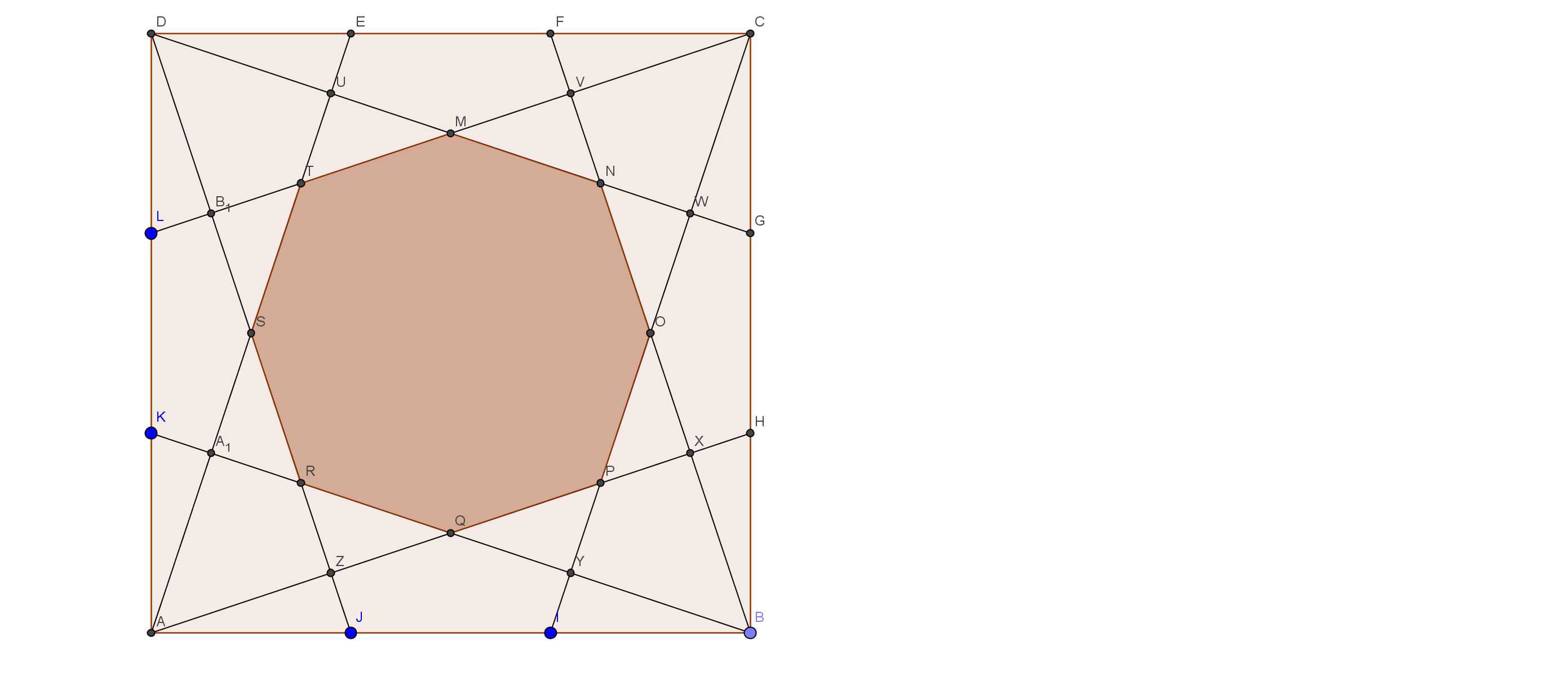# Midpoint? What About Thirds? - Part 2

Geometry Level 5Just like in Part 1, $ABCD$ is a square with a side length of 18, and we divide each side into three equal parts ​and mark the points $E,F,G,H,I,J,K,L$ in a clockwise manner starting from the vertex $D$. This time, we connect each of those points to the nearest vertex that is opposite to them to form a not-necessarily-regular octagon as shown in the figure above, for example, points $A$ and $E$ will be connected in this case. What is the area of this octagon?

×